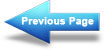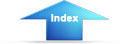Custom SearchDesign: Solar Heating of Buildings and Domestic Hot WaterMIL-HDBK-1003/13A
Worksheet D-2 is begun by selecting collector area of 200 sq ft as an
arbitrary size. Then 200 sq ft was multiplied by FI and FL from Worksheet
D-1. For each pair _ of points ACFI and ACFL, Figure 3-1 is entered
to find f.
When  f, Worksheet D-2, average yearly load carried by solar
heating, is calculated, a result of 0.646 is obtained.  QL and [SIGMA]QL
are from Worksheet C-1.  Another area of 300 sq ft is selected and found to
provide 0.794 of the load.  A smaller area could have been evaluated to
provide the DHW load only.  This information is transferred to Worksheet E-1.
Storage is selected on the basis of guidelines given in Section 2.2 (1.8
gal/ft2) to give 360 gal and 540 gal for the two collector areas
investigated.
Use Worksheet E-1 to determine value of fuel saved.  The present worth is
calculated from column (6) or (7) of Worksheet E-1 (see Section 3.7.3 for
explanation of which to use).  For this example, the no discount method,
natural gas fuel, and appropriate inflation factors (Table 3-5b) are used.
O&M costs are set equal to zero.  Therefore columns (6) and (8) of Worksheet
E-1 are not used.  Worksheet E-2 is not used because the single present worth
factor is used as explained in Section 3.7.3.2.  Assume project starts in FY
83.  The factor 1.14 escalates the fuel saved from FY 83 to FY 84 at 14% per
year (per Section 3.7.1).  That leaves 24 years at 8% inflation for which the
present worth factor is 72.106 (from Table 3-5b).  Column (7) of Worksheet
E-1 can now be calculated as 147.13 x 1.14 x 72.106 for 200 sq ft.
Fluid temperature rise through the collector is calculated on Worksheet E-1
for a selected month.  Calculation may be repeated for each month desired.
For the month of December and 200 sq ft collector area, f is 0.53, QL is
8.72 x 106 Btu/mo, G is 10 lb/hr ft2.  Using the formula on Worksheet
E-1, a temperature rise of 12.4 deg. F is found.  This is the order of
magnitude temperature rise most desired.  Note that, using the same formula,
the [DELTA]T could be fixed at, say 10 deg. F, and a flowrate, G, calculated.
Sizing of pump, pipe, and collector tube diameters for low pressure drop is
based on flowrate G (see Section 2.9).
Worksheet F may be used to calculate total installed solar system cost/sq ft,
or, the manufacturer's price for complete systems, installed, based on per
square foot of collector area, may be used.  On Worksheet F, collector cost
may be obtained from Table 3-7, Table 2-6, or manufacturer's quote may be
used.  Tank volume is based on 1.8 gal/ft2 collector for space heating/DHW.
The installed price of the insulated tank was taken from Table 2-8 (here
\$3.50/gal).  Up to 5 gal/ft2 may be specified for space heating/DHW.  DHW-
only storage may equal one or more days expected consumption.  Other costs
may be priced from a detailed design or figures from Table 3-6 may be used.
Other costs listed on Worksheet G are neglected for this example for
simplicity.
Worksheet A may now be filled in with the values from the various worksheets.
When this is done the solar system using 200 sq ft of collector is found to
be cost effective in that it saves more in fuel than it costs to install.
The 300 sq ft system is less cost effective.  This fact may also be seen from
Worksheet D-2 for the 300 sq ft system. _  There are 4 months where the
fraction  of  load  supplied by  solar, f, is  equal to 1.0 and some of the
other months are close to 1.0.  Much of this system is not being utilized;
that is, it does not result in fuel savings for those months when more solar
energy is available than can be used.
131Integrated Publishing, Inc. - A (SDVOSB) Service Disabled Veteran Owned Small Business